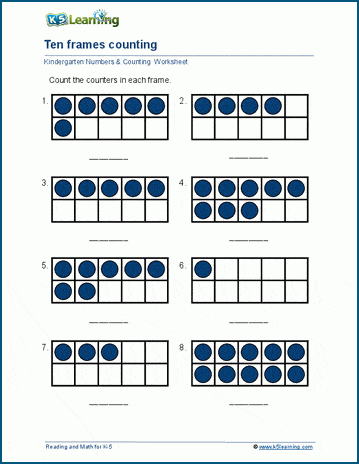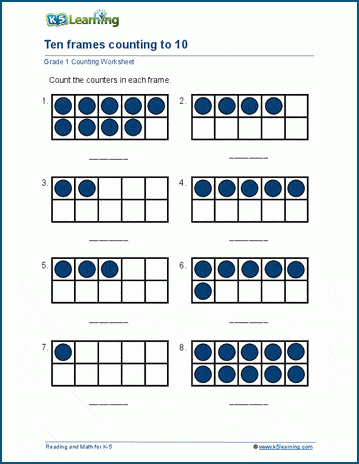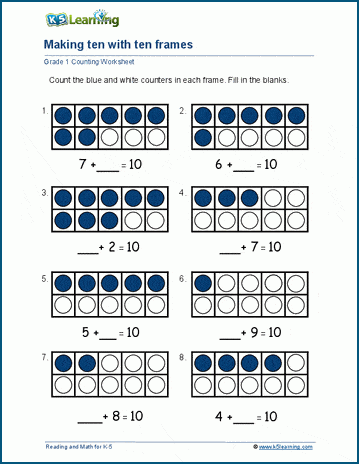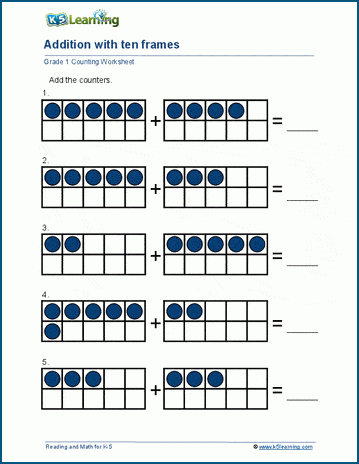# Ten Frame Worksheets for Kindergarten and Grade 1

We have created sets of ten frames worksheets for kindergarten students to practice basic number facts, and for grade 1 students to practice sums to 10 and sums to 20 using ten frames.

Ten frames help students develop basic number sense through the composition and decomposition of numbers. It’s a great visual aid for students to understand counting, addition and subtraction and a great preparation for practicing mental math.

## Kindergarten ten frame worksheets

The worksheets in this section help young students learn numbers in the base 10 counting system. There are worksheets for counting units, and worksheets for drawing counters. A blank template worksheet is also provided for teachers and parents to reuse as required.## Counting to 10 and 20 with ten frame worksheets

The first set of grade 1 worksheets has students work on counting to 10, then counting to 20 and finally drawing counters.## Simple addition using ten frames

In these ten frame worksheets, students learn the complements of 10 by adding numbers that make 10. In the first set of worksheets, one addend is missing and in the second set both addends are missing.